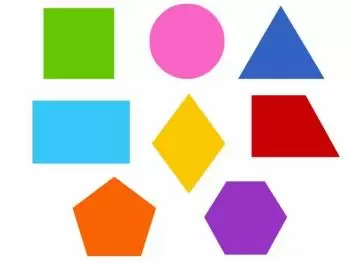# Geometric Flat Shapes: Characteristics and TypesGeometric shapes are fundamental elements in mathematics and play a crucial role in our daily lives.

From the first strokes in the sand by our ancestors to the complex applications in modern architecture, geometric shapes have been a constant source of inspiration and utility.

## What Are Geometric Figures?

Geometric figures are shapes that can be defined by specific mathematical properties. These properties include lengths, angles, areas and volumes, which allow a precise and rigorous description of each figure.

These shapes can be two-dimensional or three-dimensional. However, when they are referred to simply as unspecified "geometric shapes", we are generally referring to two-dimensional figures, which are flat and have only length and width. Three-dimensional shapes, on the other hand, have length, width and height, occupying space in three dimensions.

## Basic Geometric Shapes: What They Are and Definition

Geometric shapes are made up of a composition of basic geometric elements. Basic geometric figures are the simplest and most fundamental geometric shapes in the study of geometry. These figures serve as the essential building blocks for understanding more advanced concepts and solving geometric problems.

In this section you will find what these elements are with a small definition.

### Point

The point is the fundamental element of any plane figure. Despite its smallness, it is used as a basis for constructing a variety of shapes on a plane. In fact, the point is the cornerstone of all geometric constructions, regardless of their complexity.

### Straight Line

The straight line lies completely in the same plane. It is composed of an infinite number of points arranged in an infinite path without limits or borders. Straight lines are essential in geometry and are used as a basis for constructing other shapes.

### Vertex

A vertex is the meeting point where two straight lines intersect in a geometric figure. Vertices are essential for defining the shape and properties of many geometric figures.

### Angle

An angle is formed when two rays (called sides) share a common point of origin (vertex). Angles are measured in degrees and are used to describe turns and directions.

A quadrilateral is a flat geometric figure that has four edges and four vertices. Examples of common quadrilaterals include the square, rectangle, parallelogram, rhombus, and trapezoid. Each of these quadrilaterals has unique properties and characteristics.

### Polygon

A polygon is a geometric figure composed of three or more straight lines that join at vertices to form a closed shape. Polygons are an essential component in geometry and can vary in shape and number of sides, allowing them to represent a wide range of figures on the plane.

## Examples of Flat Geometric Shapes

Some of the most basic geometric shapes include:

### 1. Circle

The circle is a geometric figure that consists of all points that are at a constant distance, called the radius, from a central point called the center. Circles are known for their symmetry and are widely used in geometry and trigonometry.

#### Applications

• In engineering, circles are used to design wheels and gears.

• In mathematics, they are fundamental for the study of analytical geometry.

• In art, circles can be a source of inspiration for creating patterns and designs.

### 2. Triangle

The triangle is a geometric figure that has three sides and three angles. Triangles are classified into different types according to the length of their sides and the measurement of their angles.

#### Applications

• In architecture, equilateral triangles are used in the construction of stable structures.

• In navigation, triangles are used in trigonometry to calculate distances and directions.

• In geometry, triangles are the basis of many theorems and concepts.

### 3. Rectangle

A rectangle is a quadrilateral with four right angles (90 degrees) and opposite sides of equal length. It is a common geometric figure in everyday life.

#### Applications

• In architecture, rectangles are the basis of many structures, such as windows and doors.

• In mathematics, they are used to calculate areas and perimeters of rectangular surfaces.

• In graphic design, rectangles are useful for creating frames and page layouts.

### 4. Square

A square is a special type of rectangle in which all sides are of equal length. It has four right angles.

#### Applications

• In construction, squares are essential for accurate alignment and measurement.

• In mathematics, squares are the basis of Euclidean geometry.

• In art, squares can be a source of inspiration for creating patterns and abstract works.

## Importance of Geometric Figures

Geometric shapes are essential in solving mathematical problems and have a wide range of practical applications in various fields. They help scientists, engineers, architects and designers understand and model the world around us.

Geometry also plays an important role in children's cognitive development, encouraging skills such as problem solving, spatial visualization, and logic.

Author:

Published: January 12, 2022
Last review: September 14, 2023